## Example Questions

← Previous 1

### Example Question #41 : Algebra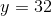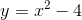Quantity A: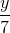Quantity B:Quantity A is greater.

Quantity B is greater.

The relationship cannot be determined from the information given.

The two quantities are equal.

The relationship cannot be determined from the information given.

Explanation:

We are given that y = 32.  Plug this value of y into the second equation.

32 = x2 – 4

36 = x2

x = +/– 6.

Next find a value for Quantity A:

y/7 = 32/7

This number is less than +6, but more than –6. Thus, the relationship cannot be determined from the information given.

### Example Question #1 : Equations / Inequalities

Column A: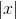Column B: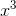The values are equal.

Column B is greater.

Column A is greater.

The relationship cannot be determined.

The relationship cannot be determined.

Explanation:

Column B is greater for positive numbers.

The columns are equal for 0.

Column A is greater for negative numbers.

Because our answer changes depending on the value inserted, we cannot determine the relationship.

### Example Question #131 : Linear / Rational / Variable Equations

Find the solution to the following equation if x = 3:

y = (4x2 - 2)/(9 - x2)

6

0

3

no possible solution

no possible solution

Explanation:

Substituting 3 in for x, you will get 0 in the denominator of the fraction. It is not possible to have 0 be the denominator for a fraction so there is no possible solution to this equation.

### Example Question #1 : How To Find Out When An Equation Has No Solution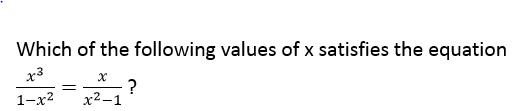I.  x = 0

II. x = –1

III. x = 1

I only

I, II, and III

II only

II and III only

III only

I only

Explanation: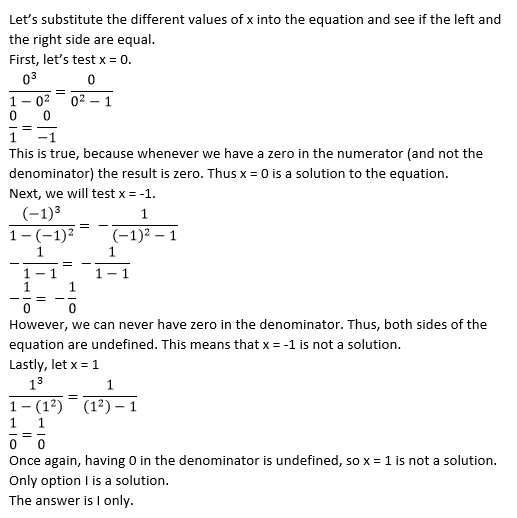### Example Question #1 : Equations / Inequalities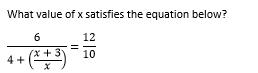1

–1/2

–3

There is no solution

3

There is no solution

Explanation: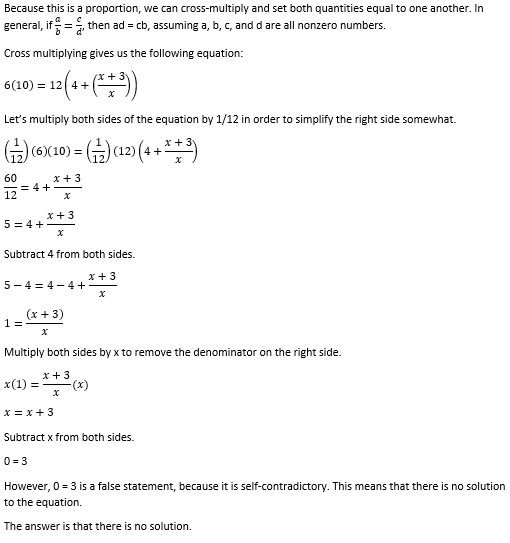### Example Question #42 : Gre Quantitative Reasoning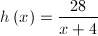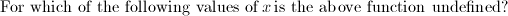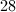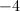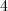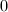Explanation:

A fraction is considered undefined when the denominator equals 0. Set the denominator equal to zero and solve for the variable.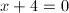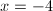### Example Question #1 : How To Find Out When An Equation Has No Solution

Solve: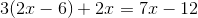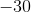Explanation:

First, distribute, making sure to watch for negatives.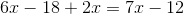Combine like terms.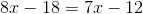Subtract 7x from both sides.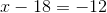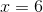### Example Question #44 : Algebra

Solve: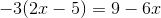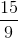Infinitely Many SolutionsNo Solution

No Solution

Explanation:

First, distribute theto the terms inside the parentheses.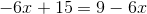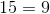This is false for any value of. Thus, there is no solution.

### Example Question #45 : Algebra

Solve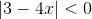.

No solutions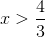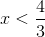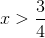No solutions

Explanation:

By definition, the absolute value of an expression can never be less than 0. Therefore, there are no solutions to the above expression.

### Example Question #2 : Equations / Inequalities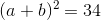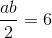Quantity A: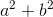Quantity B: 11

Quantity B is greater

The two quantities are equal.

Quantity A is greater

The relationship cannot be determined.

Quantity B is greater

Explanation:

Expand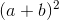out into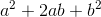.

Since, it can be seen that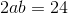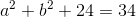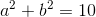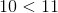so Quantity B is greater.

← Previous 1

### All GRE Math Resources